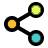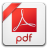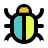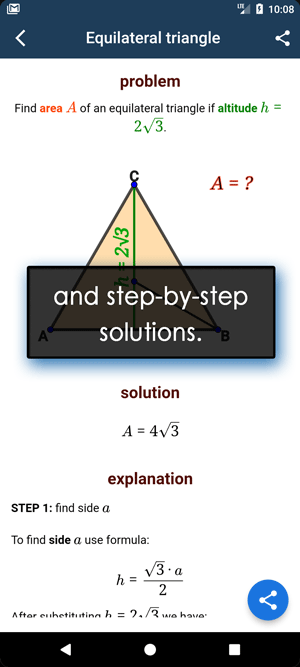Math Calculators, Lessons and Formulas

It is time to solve your math problem

mathportal.org

# Polynomial roots calculator

This free math tool finds the roots (zeros) of a given polynomial. The calculator computes exact solutions for quadratic, cubic, and quartic equations.
It also displays the step-by-step solution with a detailed explanation.

problem

Find roots of polynomial $$p(x) = 2x^3-4x^2-3x+6$$

solution

The roots of polynomial $p(x)$ are:

\begin{aligned}x_1 &= 2\\[1 em]x_2 &= \frac{\sqrt{ 6 }}{ 2 }\\[1 em]x_3 &= - \frac{\sqrt{ 6 }}{ 2 } \end{aligned}

explanation

Step 1:

Use rational root test to find out that the $\color{blue}{ x = 2 }$ is a root of polynomial $2x^3-4x^2-3x+6$.

The Rational Root Theorem tells us that if the polynomial has a rational zero then it must be a fraction $\dfrac{ \color{blue}{p}}{ \color{red}{q} }$, where $p$ is a factor of the constant term and $q$ is a factor of the leading coefficient.

The constant term is $\color{blue}{ 6 }$, with factors of 1, 2, 3 and 6.

The leading coefficient is $\color{red}{ 2 }$, with factors of 1 and 2.

The POSSIBLE zeroes are:

\begin{aligned} \dfrac{\color{blue}{p}}{\color{red}{p}} = & \dfrac{ \text{ factors of 6 }}{\text{ factors of 2 }} = \pm \dfrac{\text{ ( 1, 2, 3, 6 ) }}{\text{ ( 1, 2 ) }} = \\[1 em] = & \pm \frac{ 1}{ 1} \pm \frac{ 2}{ 1} \pm \frac{ 3}{ 1} \pm \frac{ 6}{ 1} ~~ \pm \frac{ 1}{ 2} \pm \frac{ 2}{ 2} \pm \frac{ 3}{ 2} \pm \frac{ 6}{ 2} ~~ \end{aligned}

Substitute the possible roots one by one into the polynomial to find the actual roots. Start first with the whole numbers.

We can see that $p\left( 2 \right) = 0$ so $x = 2$ is a root of a polynomial $p(x)$.

To find remaining zeros we use Factor Theorem. This theorem states that if $\dfrac{p}{q}$ is root of the polynomial then the polynomial can be divided by $\color{blue}{qx − p}$. In this example we divide polynomial $p$ by $\color{blue}{ x-2 }$

$$\frac{ 2x^3-4x^2-3x+6}{ x-2} = 2x^2-3$$

Step 2:

The solutions of $2x^2-3 = 0$ are: $x = - \dfrac{\sqrt{ 6 }}{ 2 } ~ \text{and} ~ x = \dfrac{\sqrt{ 6 }}{ 2 }$.

You can use step-by-step quadratic equation solver to see a detailed explanation on how to solve this quadratic equation.

## Report an Error !

Script name : polynomial-roots-calculator

Form values: 2x^3-4x^2-3x+6 , g , Find roots of 2x^3-4x^2-3x+6 - mathportal ,

Comment (optional)

## Share Result

Or just copy and paste the link wherever you need it.Polynomial Roots Calculator
find real and complex zeros of a polynomial
show help ↓↓ examples ↓↓ tutorial ↓↓
x^2 - 4x + 3
2x^2 - 3x + 1
x^3 – 2x^2 – x + 2
 display polynomial graphworking...
EXAMPLES
example 1:ex 1:
find roots of the polynomial $4x^2 - 10x + 4$
example 2:ex 2:
find polynomial roots $-2x^4 - x^3 + 189$
example 3:ex 3:
solve equation $6x^3 - 25x^2 + 2x + 8 = 0$
example 4:ex 4:
find polynomial roots $2x^3-x^2-x-3$
example 5:ex 5:
find roots $2x^5-x^4-14x^3-6x^2+24x+40$
Search our database of more than 200 calculators
TUTORIAL

## How to find polynomial roots ?

The process of finding polynomial roots depends on its degree. The degree is the largest exponent in the polynomial. For example, the degree of polynomial $p(x) = 8x^\color{red}{2} + 3x -1$ is $\color{red}{2}$.

We name polynomials according to their degree. For us, the most interesting ones are: quadratic - degree 2, Cubic - degree 3, and Quartic - degree 4.

This is the standard form of a quadratic equation

$$a\,x^2 + b\,x + c = 0$$

The formula for the roots is

$$x_1, x_2 = \dfrac{-b \pm \sqrt{b^2-4ac}}{2a}$$

Example 01: Solve the equation $2x^2 + 3x - 14 = 0$

In this case we have $a = 2, b = 3 , c = -14$, so the roots are:

\begin{aligned} x_1, x_2 &= \dfrac{-b \pm \sqrt{b^2-4ac}}{2a} \\ x_1, x_2 &= \dfrac{-3 \pm \sqrt{3^2-4 \cdot 2 \cdot (-14)}}{2\cdot2} \\ x_1, x_2 &= \dfrac{-3 \pm \sqrt{9 + 4 \cdot 2 \cdot 14}}{4} \\ x_1, x_2 &= \dfrac{-3 \pm \sqrt{121}}{4} \\ x_1, x_2 &= \dfrac{-3 \pm 11}{4} \\ x_1 &= \dfrac{-3 + 11}{4} = \dfrac{8}{4} = 2 \\ x_2 &= \dfrac{-3 - 11}{4} = \dfrac{-14}{4} = -\dfrac{7}{2} \end{aligned}

### Quadratic equation - special cases

Sometimes, it is much easier not to use a formula for finding the roots of a quadratic equation.

Example 02: Solve the equation $2x^2 + 3x = 0$

Because our equation now only has two terms, we can apply factoring. Using factoring we can reduce an original equation to two simple equations.

\begin{aligned} 2x^2 + 3x &= 0 \\ \color{red}{x} \cdot \left( \color{blue}{2x + 3} \right) &= 0 \\ \color{red}{x = 0} \,\,\, \color{blue}{2x + 3} & \color{blue}{= 0} \\ \color{blue}{2x } & \color{blue}{= -3} \\ \color{blue}{x} &\color{blue}{= -\frac{3}{2}} \end{aligned}

Example 03: Solve equation $2x^2 - 10 = 0$

This is also a quadratic equation that can be solved without using a quadratic formula.

. \begin{aligned} 2x^2 - 18 &= 0 \\ 2x^2 &= 18 \\ x^2 &= 9 \\ \end{aligned}

The last equation actually has two solutions. The first one is obvious

$$\color{blue}{x_1 = \sqrt{9} = 3}$$

and the second one is

$$\color{blue}{x_2 = -\sqrt{9} = -3 }$$

### Roots of cubic polynomial

To solve a cubic equation, the best strategy is to guess one of three roots.

Example 04: Solve the equation $2x^3 - 4x^2 - 3x + 6 = 0$.

Step 1: Guess one root.

The good candidates for solutions are factors of the last coefficient in the equation. In this example, the last number is -6 so our guesses are

1, 2, 3, 6, -1, -2, -3 and -6

if we plug in $\color{blue}{x = 2}$ into the equation we get,

$$2 \cdot \color{blue}{2}^3 - 4 \cdot \color{blue}{2}^2 - 3 \cdot \color{blue}{2} + 6 = 2 \cdot 8 - 4 \cdot 4 - 6 - 6 = 0$$

So, $\color{blue}{x = 2}$ is the root of the equation. Now we have to divide polynomial with $\color{red}{x - \text{ROOT}}$

In this case we divide $2x^3 - x^2 - 3x - 6$ by $\color{red}{x - 2}$.

$$( 2x^3 - 4x^2 - 3x + 6 ) \div (x - 2) = 2x^2 - 3$$

Now we use $2x^2 - 3$ to find remaining roots

\begin{aligned} 2x^2 - 3 &= 0 \\ 2x^2 &= 3 \\ x^2 &= \frac{3}{2} \\ x_1 & = \sqrt{ \frac{3}{2} } = \frac{\sqrt{6}}{2}\\ x_2 & = -\sqrt{ \frac{3}{2} } = - \frac{\sqrt{6}}{2} \end{aligned}

### Cubic polynomial - factoring method

To solve cubic equations, we usually use the factoting method:

Example 05: Solve equation $2x^3 - 4x^2 - 3x + 6 = 0$.

Notice that a cubic polynomial has four terms, and the most common factoring method for such polynomials is factoring by grouping.

\begin{aligned} 2x^3 - 4x^2 - 3x + 6 &= \color{blue}{2x^3-4x^2} \color{red}{-3x + 6} = \\ &= \color{blue}{2x^2(x-2)} \color{red}{-3(x-2)} = \\ &= (x-2)(2x^2 - 3) \end{aligned}

Now we can split our equation into two, which are much easier to solve. The first one is $x - 2 = 0$ with a solution $x = 2$, and the second one is $2x^2 - 3 = 0$.

\begin{aligned} 2x^2 - 3 &= 0 \\ x^2 = \frac{3}{2} \\ x_1x_2 = \pm \sqrt{\frac{3}{2}} \end{aligned}